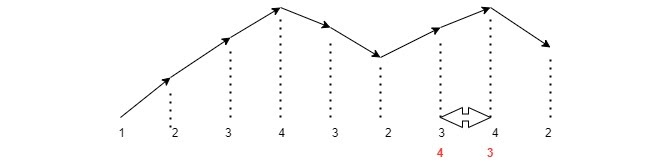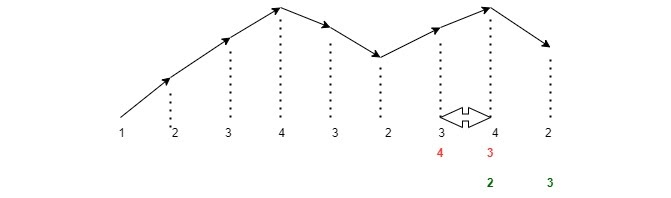# Next Permutation

## Introduction

In this blog, we will discuss a medium level array problem frequently asked in Interviews.

The problem is to find the Next Permutation of a number, which is the next lexicographical permutation of the number, by rearranging the digits.

We are given an N digit number, and the goal is to find the next greater permutation of a number.

Example:

Let the number be 1243. Its next permutation will be 1324.

Let the number be 1241. Its next permutation will be 1412.

Say for number 132.

All possible permutations arranged in lexicographical order would be:

123

132

213 ↩

231

312

321

For 132, our answer would be 213.

To explain in detail, say the number given is 123432342.

To find its next permutation, We first drew this graph. Now shifting any peak towards the left would give higher permutations, but it doesn’t give the next higher permutation.

So, we swap using the last ascending subarray to get the exact next higher permutation because any element before it will have a higher weightage.

∴We are swapping element near the highest peak, where we encounter the first decreasing sequence, with the immediate highest number in the remaining array and having lesser weight elements.

Here, point of change = 3

And, Point for substitution = 4.To get the smallest possible permutation, after we swap the elements, we sort the numbers beginning from the switched part in ascending order because we already have a more significant number because of swapping.The required element is 123432423.

Note: A particular case exists. Suppose elements present are in descending order. Then we just need to reverse the number. (Why?) This is because it is the last number present in order and next to that, we will have the first number present.

## Approach for the problem

The number starts changing from the first decreasing sequence from the end. Therefore, find the point of change first.

Find the number for substitution. It would be the next highest number in the remaining array.

Swap point of change and number for substitution.

Rearrange remaining numbers to make it minimum. Sort/Reverse.

We get the desired next Permutation.∴Point of change=1.

The number for substitution=4.

Here, our number was 11541. On swapping, we get 14511.

After sorting the remaining numbers in 14511, we get 14115.

Till now, I guess you must have got the basic idea of what has been asked in the problem statement. So, I strongly recommend you first give it a try.

https://www.codingninjas.com/codestudio/problem-details/next-permutaion_893046

If you were unable to solve it, don’t be sad.

Please have a look at the algorithm, and then again, you must give it a try.

### PseudoCode

Algorithm

___________________________________________________________________

procedure nextPermutation( ):

___________________________________________________________________

1.  n=nums.size(); index=-1

2.  for n-1 to 0.

3.   if nums[i]>nums[i-1]

4.   Get the index and give a break command to come out of the loop.

5.   if index==-1.

6.   Return reverse of the number.

7.   Inside the else statement, find the number for substitution.

8.   for index+1 to n.

9.   Check nums[i]>nums[index-1] and nums[i]<=nums[previous]. Update previous with new index.

10.  Swap nums[index-1] and nums[previous]

11. Rearrange remaining numbers by sorting or reversing.

12. end procedure

___________________________________________________________________

## Implementation in C++

Now, let’s have a look at the Code:

``````class Solution {
public:
void nextPermutation(vector<int>& nums) {
int index=-1;
int n=nums.size();

//finding point of change.

for(int i=n-1; i>0; i--){
if(nums[i]>nums[i-1]){
index=i;
break;
}
}

//If there is no point of change, then this is a special case. As discussed above, just reverse the number.

if(index==-1){
reverse(nums.begin(),nums.end());
}

else{
int previous=index;

//   Finding number for substitution.
for(int i=index+1; i<n; i++){
if(nums[i]>nums[index-1] && nums[i]<=nums[previous]){
previous=i;
}
}

//Swapping point of change and number for substitution.
swap(nums[index-1],nums[previous]);

//Rearranging remaining numbers.
reverse(nums.begin()+index, nums.end());
}

}
};``````

Output

``````Sample Input:
123

Sample Output:
132``````

### Complexity Analysis

Time Complexity: O(n).

Analysing Time Complexity:

To find point of change: O(n) time + To find number for substitution: O(n) time + To swap: O(1) time + To reverse: O(n/2) time.

∴ Total time = O(n).

By the Brute-force method, it would have taken O(n！) time.

Space complexity: O(1) since no extra space is required.

1. How to approach a problem like this?
Answer) First, understand the problem. The best way is to use diagrams. Then write your algorithm. Then start with the Brute Force method. Lastly, try to optimise your code.

2. Is there any Prerequisite for this problem?
Answer)Yes, you must understand what is lexicographical order and arrange something lexicographically. Then this problem would seem easy.

3. Is there any shortcut for this problem?
Answer)Yes, in C++ STL, there exists a predefined function called next-permutation. If the implementation is not asked, feel free to use that.

## Key Takeaways

This article taught us how to find the next permutation of a number. It rearranges numbers into the lexicographically next greater permutation of numbers. We discussed its implementation using illustrations, pseudocode, and then proper code.

We hope you could take away critical techniques like analyzing problems by walking over the execution of the examples and finding out the pattern followed.

Now, we recommend you practice problem sets based on this to master your fundamentals. You can get a wide range of questions similar to this on CodeStudio